Chapter 11 Work, Power and Energy Class 9 Science Important Questions with Answers PDF will help you in scoring more marks in your exams.

## Work, Power and Energy Class 9 Important Questions and Answers Science Chapter 11

Based on work done

Question 1.
Define the term ‘work done’. (CBSE 2011, 2012, 2015)
Definition: Work is said to be done by a force on a body or an object if the force applied causes a displacement in the body or the object.

More Resources

Question 2.
How much work is done by a man who tries to push the wall of a house but fails to do so.
(Similar CBSE 2011, 2013)
W = FS = 0 (∴ S = 0)

Question 3.
A body of mass 2 kg is moving in a circular path of radius 2m. How much work is done on the body ?
(CBSE 2011)
Zero. This is because centripetal force acting on the body is perpendicular to the displacement of the body (W = FS cos 90° = 0).

Question 4.
What would be the amount of work done on an object by a force, if the displacement of the object is zero ?
W = FS = 0 (∴ S = 0)

Question 5.
What is the work done by the earth in moving around the sun ? (CBSE 2011)
Zero.

Question 6.
When is work done by a force negative ? (CBSE 2011, 2012, 2014)
Work done by a force is negative, when the displacement of the body is in the direction opposite to the direction of the force.

Question 7.
When is work done by a force positive ? (CBSE 2012)
Work done by a force is positive, when the displacement of the body is in the direction of the force.

Question 8.
When is work done by a force zero ? (CBSE 2011)
Work done by a force is zero, when body is not displaced from its position by the force or when force acting on the body is perpendicular to the direction of the displacement of the body.

Question 9.
Write two conditions under which work is said to be done. (CBSE 2011, 2012)

1. Force must act on the body and
2. the body must be displaced from its position either in the direction of applied force or in a direction opposite to the direction of the applied force.

Question 10.
What is SI unit of work ? (CBSE 2015)
S.I. unit of work is joule (J).

Question 11.
Write the expression for a work done by a force F acting on an object at an angle θ with the displacement S of the object.
W = FS cos θ.

Question 12.
What should be the angle between force and displacement for maximum work ? (CBSE 2013)
W = FS cos θ. Work will be maximum if cos θ = 1 or θ = 0°.

Question 13.
Does the work done depend upon how fast an object is raised ? Explain. (CBSE 2013)
Work done depends on the force applied and distance travelled by an object. It does not depend on how
fast an object is raised.

Based on energy (Kinetic energy and Potential energy)

Question 14.
Define the “energy”.
The capacity of doing work by an object is known as the energy of the object.

Question 15.
What is SI unit of energy ?
S.I. unit of energy is joule (J).

Question 16.
Define mechanical energy.

Or

Name two forms of mechanical energy.
Mechanical energy is the sum of Kinetic energy and potential energy.

Question 17.
Define Kinetic energy.
The energy possessed by a body by virtue of its motion is called kinetic energy..

Question 18.
What is SI unit of kinetic energy ? Define it.
joule (J). Kinetic energy is said to be 1 J if a body of mass 1 kg moves with a speed of 1 ms-1.

Question 19.
What type of energy is possessed by a cricket ball just before being caught by a fielder ?
Kinetic energy.

Question 20.
What type of energy is possessed by a flowing water ?
Kinetic energy.

Question 21.
Identify the energy possessed by a rolling stone. (CBSE 2011)
Kinetic energy.

Question 22.
Identify the kind of energy possessed by a running athlete. (CBSE 2011)
Kinetic energy.

Question 23.
A car and a truck are moving with the same velocity of 60 km/hr. Which one has move kinetic energy ?
(Mass of truck > mass of car). (CBSE 2011)
K.E = 1/2 mv2. Since mass of truck is greater than the mass of car and both have same velocity, therefore, K.E. of track > K.E. of car.

Question 24.
What will be the kinetic energy of a body when its mass is made four times and the velocity is doubled ?
(CBSE 2011)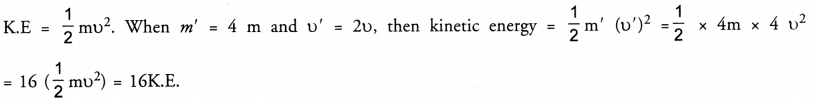Question 25.
If we lift a body of mass 7 kg vertically upwards 10 m, then calculate the force required to lift the body
(g = 10 ms-2). (CBSE 2011)
Force = mg = 7 x 10 = 70 N.

Question 26.
Define potential energy of an object.
(CBSE Sample Paper 2010, CBSE 2011, 2012, 2014, 2016, 2017)
The energy possessed by an object by virtue of its position or shape is known as potential energy.

Question 27.
Give an example of body having potential energy due to change of shape. (CBSE 2011)
Spring of a watch or stretched bow and arrow.

Question 28.
Name the type of energy possessed by a raised hammer. (CBSE 2011)
Potential energy.

Question 29.
State SI unit of potential energy. (CBSE Sample Paper 2010, CBSE 2011, 2014, 2016)
joule (J)

Question 30.
Give an example of an object having elastic potential energy. (CBSE 2011)
A stretched spring or a compressed spring has elastic potential energy.

Question 31.
In what form, energy is stored in a wrist watch ?
Potential energy.

Based on transformation of energy

Question 32.
A torch cell converts one form of energy into another form. Name these two forms of energy.
(CBSE 2011)
Chemical energy is converted into electric energy.

Question 33.
A log of wood cut by a saw becomes hot. From where this heat energy comes ?
Mechanical energy is converted into heat energy.

Question 34.
When we switch on the electric bulbs, one form of energy is converted into another form. Name these forms of energy. (CBSE 2011)
Electrical energy is converted into light and heat energy.

Question 35.
When a ball is thrown vertically upwards, its velocity goes on decreasing. What happens to its potential energy as its velocity becomes zero ? (CBSE 2011)
Potential energy of the ball goes on increasing when its kinetic energy decreases. Potential energy becomes maximum and equal to initial kinetic energy when the velocity of the ball becomes zero (provided there is no air resistance).

Question 36.
Write the observed energy transformation that takes place at thermal power station. (CBSE 2011)
Chemical energy into heat energy and then to electrical energy.

Question 37.
State the transformation of energy taking place when

1. Green plants prepare their food
2. Head of a nail is hammered hard and it becomes hot. (CBSE 2011)

1. Solar energy + Green leaves —> Food (chemical energy)
2. Kinetic energy of hammer is convered into the heat energy (i.e. K.E. —> Heat energy)

Based on conservation of energy

Question 38.
State the law of conservation of energy. (CBSE 2011, 2012)
Energy can neither be created nor be destroyed but can be changed from one form into another form.

Based on power and commercial unit of energy

Question 39.
State the physical quantity which will be affected by changing the rate of doing work. (CBSE 2013)
Power ( = rate of doing work).

Question 40.
Establish a relationship between SI unit and commercial unit of energy.
Or

Derive the relation between the commercial unit of energy and joules.
SI unit of energy is joule and commençai unit of energy is kWh.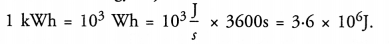Question 41.
Name the commercial unit of electric energy.
The commercial unit of electric energy is kilowatt hour (kWh).

Question 42.
State the relation between commercial unit of energy and joule.

Or

How many joules make one kilowatt hour ?
Commercial unit of energy is kWh. 1 kWh = 3.6 x 106 J.

Based on work done

Question 1.
Distinguish between positive and negative work. When you lift an object, two forces act on it. Identify these forces. Which one of the two does,

1. positive work

Work is said be positive if the body is displaced in the direction of the applied force. Work is said to be negative if the body is displaced in a direction opposite to the direction of applied force.
When we lift an object, the forces acting on the object are

1. force of gravity and
2. muscular force.

Force of gravity does negative work as force of gravity acts downwards and the object is displaced upwards. Muscular force does positive work as the object is displaced in the direction of the applied muscular force.

Question 2.
A boy throws a rubber ball vertically upwards. What kind of work is done;

1. by the force applied by the boy,
2. by the gravitational force. Support your answer with reason. (CBSE 2011, 2013)

1. Work done by the force applied by the boy is positive as both the applied force and the displacement of the ball are in the same direction.
2. Work done by gravitational force is negative as the force and the displacement of the ball are in opposite direction.

Question 3.
A coolie holding a heavy box is waiting for someone at the bus stand for 15 minutes. How much work has been done by him ? (Similar CBSE 2011)

Or

A person holds a suitcase for 30 minutes and gets tired. Has he done some work or not ? Justify your answer.
(CBSE 2012)
Since coolie is applying force on the heavy box to hold it but there is no displacement of the box (i.e. S = 0), therefore, work done by him is zero (∴ W = FS cos θ = 0).

Question 4.
Work done by a coolie on the luggage on his head while carrying it on horizontal road. (CBSE 2013)
The force of gravity acting on the box acts vertically downward and the displacement is in the horizontal direction, so θ = 90°. Hence, W = FS cos θ = FS cos 90° = 0

Question 5.
In a tug of war, one team wins and the other team loses. Which team does positive work and which one does negative work ? Justify your answer. (CBSE 2011)
Winning team does positive work on the rope because the force applied by the team on the rope acts in the direction of the displacement of the rope. On the other hand, losing team does negative work as the force applied by the team on the rope acts in the direction opposite to the direction of the displacement of the rope.

Based on Kinetic and potential energies

Question 6.
Establish the relationship between kinetic energy and momentum of body. (CBSE 2012)
Relationship between kinetic energy and momentum of body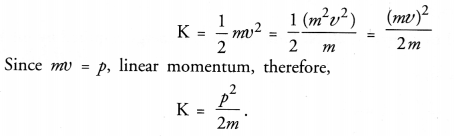Question 7.
Which would have greater effect on the kinetic energy of an object-doubling the mass or doubling the velocity ? (CBSE 2011
K.E. = 1/2 mv2 . When mass is doubled, K.E. becomes 2 times its initial value. When velocity is doubled,
K.E. becomes 4 times its initial value. Thus, doubling the velocity would have greater effect on the kinetic energy than doubling the mass.

Question 8.
A bus and a car have same kinetic energy. Which of the two is moving fast ? Explain. (CBSE 2011)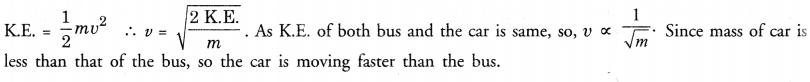Question 9.
Is it possible that an object is in the state of accelerated motion due to external force acting on it, but no work is being done by the force. Explain it with an example. (NCERT Question Bank, CBSE 2012)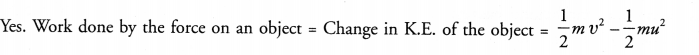When an object has uniform circular motion, the speed of the object remains the same i.e. v = u. Hence, work done by the force = zero.

Question 10.
The velocity of a body moving in a straight line is increased by applying a constant force F, for some distance in the direction of the motion. Prove that the increase in the kinetic energy of the body is equal to the work done by the force on the body. (CBSE 2011)
Consider a body or an object of mass m moving with velocity u. Let a force F be applied on the body so that the velocity attained by the body after travelling a distance S is v (Figure 5).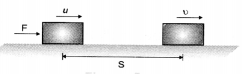Work done by the force on the body is given by
W =      FS                                                                               …(i)
Since velocity of the body changes so the body is accelerated. Let a be the acceleration of the body. Therefore, according to Newton’s second law of motion,
F =     ma                                                                             …(ii)
Using eqn. (ii) in eqn. (i), we get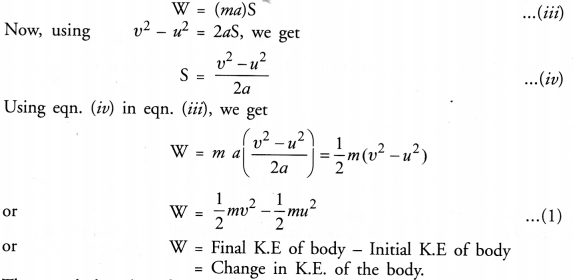Thus, work done by a force on a body is equal to the change in kinetic energy of the body This is known as work-energy theorem.

Question 11.
Two bodies of same mass start from rest and move with velocities of V and 2V respectively. Find the ratio of their kinetic energies. (CBSE 2012)Question 12.
Two bodies of masses my and m2 have equal kinetic energies. What is the ratio of their linear momenta ?
(CBSE 2012)

Or

Two balls have different masses but same kinetic energy. Which has the greater momentum ? Justify.
(CBSE 2013)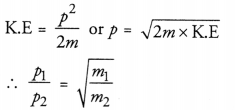Thus, momentum of the ball of large mass is more.

Question 13.
Derive an expression for the potential energy of an object of mass m that has been raised to a height ‘h’ from the ground. (CBSE 2011, 2014, 2016)

Or

Derive an expression for potential energy.
The potential energy stored in an object due to its vertical position with respect to the surface of the earth is called gravitational potential energy.
Gravitational potential energy of an object at a height h above the surface of earth is equal to the work done in raising the object through a height h against the gravitational force or gravity.
Consider an object of mass m raised to a height h against gravity (Figure 7).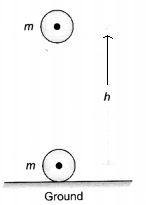Minimum force required to lift the object, F = weight of body = mg
Distance travelled by the object, S = h
∴                        Work done, W = F x S = mgh
This work done against force of gravity is equal to the potential energy or gravitational potential energy of the object. i. e., P.E. = mgh
Thus, gravitational potential energy of an object depends upon

1. the weight (mg) of the object and
2. the height (h) of the object from the surface of the earth.
SI Unit of potential energy is joule (J).

Question 14.
An object is dropped from a height h when is its

1. Potential energy maximum,
2. Kinetic energy maximum. (CBSE 2011)

1. Potential energy of the object is maximum, when it is at heigh h.
2. Kinetic energy of the object is maximum, when it just touches the ground.

Based on transformation of energy

Question 15.
The head of a nail becomes warm when it is hammered into a plank of wood. Explain stating the scries of energy transformations taking place. (CBSE 2011)
The potential energy of a raised hammer is converted into the kinetic energy when hammer falls down. Then this kinetic energy of the falling hammer is converted^hto heat energy when it strikes the head of a nail. Therefore, the head of the nail becomes warm.

Question 16.
Name the devices which

1. convert chemical energy into electrical energy and
2. light energy into electrical energy.

1. A thermal power plant
2. A photocell.

Question 17.
Name the appliances which work under the following energy transformation.

1. Sound energy into electrical energy.
2. Heat energy into mechanical energy. (CBSE 2011)

1. Microphone
2. Heat engine.

Or

Name the appliances which work under the following energy’ transformation :

1. Mechanical energy into electrical energy
2. electrical energy into mechanical energy.

1. Electric generator
2. Electric motor.

Question 18.
When a body falls freely, its potential energy is converted into kinetic energy. But when the freely falling body reaches the ground, it comes to rest. What happens to the kinetic energy of the body when it stops on reaching the ground ? (CBSE 2011)
When a freely falling body stops on reaching the ground, sound is heard and the ground becomes hot, where the body strikes. So kinetic energy of a freely falling body is converted into sound energy and heat energy. Based on power and commercial unit of energy

Question 19.
A boy and a girl do the same work in 5 minutes and 10 minutes respectively. Which of these two has more power and why ?
P = W/t. As the boy takes less time to do the same work as compared to the girl, so power of the boy will be more than that of the girl.

Question 20.
Give mathematical relation between power, force and velocity. (CBSE 2011)
P = Fv.

Question 21.
Express Kilowatt in terms of joules per second. (CBSE 2012)
1 kilowatt = 1000 W
Since 1 W = 1 J s-1
∴ 1 kilowatt = 1000 J s-1

Question 22.
Differentiate between kilowatt and kilowatt hour. (CBSE 2011, 2012, 2014)
Kilowatt is the unit of power. 1 kilowatt = 1000 J s-1
Kilowatt hour is the unit of electric energy. 1 kilowatt hour = 3.6 x 106 J

Question 23.
In a house 3 bulbs of 25 W each are used for 5 hours a day. Calculate the units of electricity consumed in a month of 31 days. Also, find the total expenditure if 1 unit of electricity costs Rs. 2.50. (CBSE 2014)
Electric energy consumed by 3 bulbs in a day = power x time = 3x 25 x5 = 375 Wh
Electricity consumed in 31 days = 375 x 31 = 11625 Wh =11.625 kWh =11.625 units
Cost of electricity consumed = 11.625 x 2.50 = Rs. 29.06

Question 1.
Define the term “Work”. State the factors on which work done depends. Derive an expression for the work done by a force applied on an object at an angle 9 with the horizontal direction.

Or

Define work. State two factors on which the magnitude of work depends. (CBSE 2011)
In our daily life, the word work means any kind of mental and physical activity. For example, we say that we are doing work while

2. cooking a food.
3. walking on a level road with a box on our head.
4. pushing a wall of a house but fails to do so.

Factors on which work done depends Work done by a force depends upon :

1. The magnitude of the applied force. If a small force is applied on a body, less amount of work is done. On the other hand, if a large force is applied on the body, more amount of work is done on the body.
Thus,                     W ∝ F, where F is magnitude of applied force.
2. The distance travelled by the body on the application of force ( i. e., displacement S). If a body travels large distance on the application of force, large amount of work is done. If a body travels a small distance on the application of force, small amount of work is done.
Thus,                     W ∝ S.

Question 2.
(a) Define the term “energy”. Write the names of different forms of energy.
(b) Define kinetic energy. Derive an expression for the kinetic energy of an object of mass m moving with a velocity V. (CBSE 2011, 2017)
(a) Definition of energy : The capacity of doing work by a body or an object is known as the energy of the body or the object.
Unit of Energy: Unit of energy is same as that of the unit of work. So, SI unit of work is joule (J). When we say that energy of a body is 1 joule, it means, this body has the capacity to do 1 joule work.
The various forms of energy are :

1. Mechanical Energy: The sum of kinetic energy and potential energy of a body is known as mechanical energy.
2. Heat or Thermal Energy: The energy possessed by a body due to its temperature is known as heat or thermal energy.
3. Chemical Energy: The energy released in chemical reactions is known as chemical energy.
4. Sound Energy: The energy of a vibrating body producing sound is known as sound energy.
5. Electrical Energy: The energy of moving electrons in a conductor connected with a battery is known as electrical energy.
6. Nuclear Energy: The energy released when two nuclei of light elements combine with each other to form a heavy nucleus or when a heavy nucleus breaks into two light nuclei is known as nuclear energy.
7. Solar Energy: The energy radiated by the sun is known as solar energy.

(b) Definition : The energy possessed by a body by virtue of its motion is known as kinetic energy. In other words, we can say that anything which moves has kinetic energy.
Consider a body of mass m lying at rest on a smooth floor. Let a force F be applied on the body so that the body attains a velocity v after travelling a distance S (Figure 4).Work done by the force on the body, W = FS                                                  …(i)
Since the velocity of the body changes from zero to v, so the body is accelerated. Let a be the acceleration of the body. Then according to Newton’s second law of motion.
F = ma
Substituting the value of F = ma in eqn.(î), we get
W = (ma)S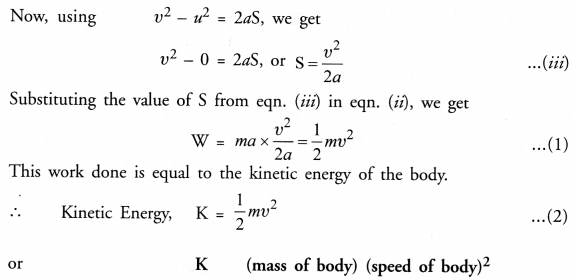K.E of a body is direcdy proportional to

1. its mass and
2. square of its speed. SI unit of kinetic energy is joule (J). Kinetic energy of a body is said to be 1 joule, if a body of 1 kg mass moves with a speed of 1 ms-2‘ Thus, 1 J = 1 kg m2s-2.

Question 3.
Define kinetic energy of an object. Show that work done by a force on an object is equal to the change in kinetic energy of the object. (CBSE 2011)
Definition : The energy possessed by a body by virtue of its motion is known as kinetic energy. In other words, we can say that anything which moves has kinetic energy.
Consider a body or an object of mass m moving with velocity u. Let a force F be applied on the body so that the velocity attained by the body after travelling a distance S is v (Figure 5).
Work done by the force on the body is given by
W =      FS                                                                               …(i)
Since velocity of the body changes so the body is accelerated. Let a be the acceleration of the body. Therefore, according to Newton’s second law of motion,
F =     ma                                                                             …(ii)
Using eqn. (ii) in eqn. (i), we get
Thus, work done by a force on a body is equal to the change in kinetic energy of the body This is known as work-energy theorem.

Question 4.
State the law of conservation of energy. Show that the energy of a freely falling body is conserved.
(CBSE 2011, 2012)

Or

State and prove the law of conservation of energy for a freely falling body. (CBSE 2012)
According to this law, “energy can neither be created nor be destroyed, but can be changed from one form to another form. ”

Or

When one form ofenergji is changed or transformed into other forms of energy, the total energy of an isolated system remains the same i. e., the total energy before transformation = the sum of the different energies transformed.
Consider a body of mass m at a height h above the ground. Suppose this position of the body is A (Figure 11).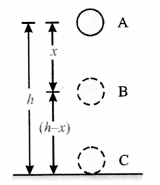Suppose the body at A is at rest i.e. v = 0.
At position A
Potential energy of the body, P.E. = mgh Kinetic energy of the body, K.E. = 0 Total energy (i.e., mechanical energy) of the body at A = P.E. + K.E. = mgh + 0 = mgh                                                       …(1)
Let the body falls freely under the action of gravity to position B through a height x. Now, the height of the body from the ground = (h-x)
At position B
Potential energy of the body, P.E. = mg (h – x)                                               ….(2)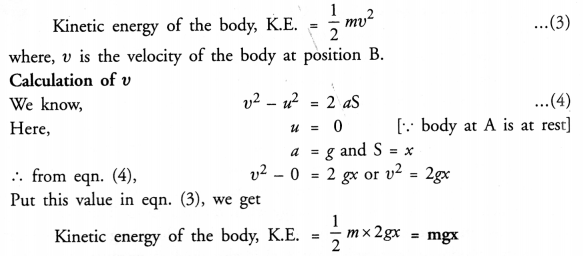Total energy (i.e., mechanical energy) of the body at B= P.E. + K.E.
= mg (h – x) + mgx = mgh – mgx + mgx = mgh      …(5)
Finally, let the body touches the ground at C, so that the distance through which it falls = h.
At position C
Potential energy of body, P.E. = mg (0) = 0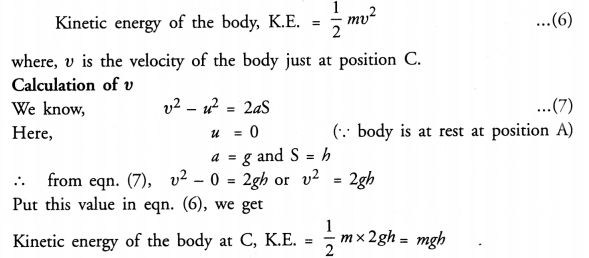Total energy (i.e., mechanical energy) of the body at C = P.E. + K.E. = 0 + mgh = mgh      …(8)
From eqns. (1), (5) and (8), it is clear that the total energy (i.e., mechanical energy) of a body at any instant during free fall of the body remains constant. Hence, the law of conservation of energy is verified.

Question 5.
Show that when a body is dropped from a certain height, the sum of its kinetic and potential energy at any instant during its fall is constant. (CBSE 2011)
Consider a body of mass m at a height h above the ground. Suppose this position of the body is A (Figure 11).Suppose the body at A is at rest i.e. v = 0.
At position A
Potential energy of the body, P.E. = mgh Kinetic energy of the body, K.E. = 0 Total energy (i.e., mechanical energy) of the body at A = P.E. + K.E. = mgh + 0 = mgh                                                       …(1)
Let the body falls freely under the action of gravity to position B through a height x. Now, the height of the body from the ground = (h-x)
At position B
Potential energy of the body, P.E. = mg (h – x)                                               ….(2)Total energy (i.e., mechanical energy) of the body at B= P.E. + K.E.
= mg (h – x) + mgx = mgh – mgx + mgx = mgh      …(5)
Finally, let the body touches the ground at C, so that the distance through which it falls = h.
At position C
Potential energy of body, P.E. = mg (0) = 0Total energy (i.e., mechanical energy) of the body at C = P.E. + K.E. = 0 + mgh = mgh      …(8)
From eqns. (1), (5) and (8), it is clear that the total energy (i.e., mechanical energy) of a body at any instant during free fall of the body remains constant. Hence, the law of conservation of energy is verified.

Hope given Previous Year Question Papers for CBSE Class 9 Science Chapter 11 Work, Power and Energy are helpful to complete your science homework.

If you have any doubts, please comment below. Learn Insta try to provide online science tutoring for you.